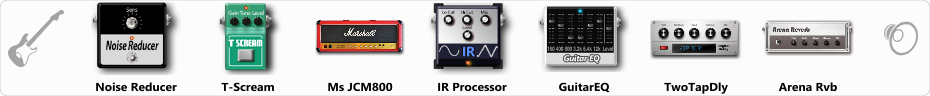Discussion in 'ToneLib-GFX presets' started by Louis_R, Oct 3, 2019.

Effects chain:Effect: "Noise Reducer" (Dynamics / Filter), active - "yes"
"Sens" = 64
"Mode" = Hard

Effect: "T-Scream" (Overdrive / Distortion), active - "yes"
"Drive" = 20
"Tone" = 100
"Level" = 100

Effect: "Ms JCM800" (Amp simulators), active - "yes"
"Gain" = 66
"Bass" = 65
"Middle" = 62
"Treble" = 64
"Presence" = 58
"Master" = 60
"Output" = 50
"Level (dB)" = 0

Effect: "IR Processor" (Dynamics / Filter), active - "yes"
"IR" = Marshall 1960A 4x12 SM57 +1_00
"Low Cut (Hz)" = 0
"Hi Cut (kHz)" = 14.0
"Mix" = 100
"Level (dB)" = 0

Effect: "GuitarEQ" (Dynamics / Filter), active - "yes"
"160 Hz" = 5
"400 Hz" = 2
"800 Hz" = 0
"1.6 kHz" = 0
"3.2 kHz" = 0
"6.4 kHz" = -3
"12 kHz" = -2
"Level (dB)" = 0

Effect: "TwoTapDly" (Delay), active - "yes"
"Time" = Note sync, 1/8 Triplet
"Feedback" = 60
"Tone" = 76
"Sens" = 0
"TapTime" = 5.0
"Mix" = 40

Effect: "Arena Rvb" (Reverb), active - "yes"
"Time" = 7.3
"PreDelay" = 23
"LoDamp" = 18
"HiDamp" = 21
"Mix" = 30

Note: You will need to download and install the ToneLib-GFX software to use the preset.

#### Attached Files:

File size:
15.1 KB
Views:
4,952
GrozzeR, NIk0lyan, anderubi and 10 others like this.
2. hola Louis, este el sonido mio con tu preset , no se si lo podras escuchar sin el impulso,#### Attached Files:

3. o impulse ja vem quando envia